heat control  r71
adc.c File Reference
`#include <math.h>`
`#include "adc.h"`
`#include "setup.h"`
Include dependency graph for adc.c: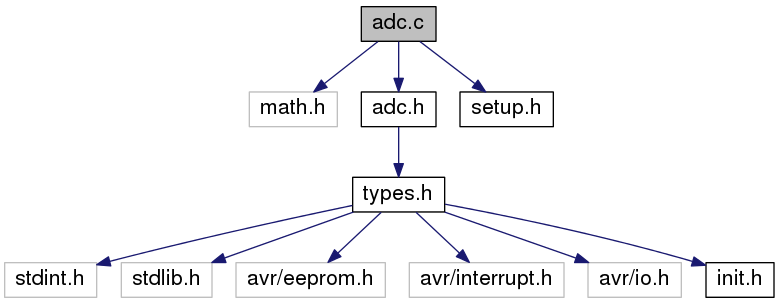Go to the source code of this file.

## Functions

void AdcInit (void)
initialize the adc resource More...

ISR (ADC_vect)
ADC interrupt service. More...

void ProcessNextConversion (void)
checks whether all converted values are processed and starts a new ADC conversion if the latter evaluates to TRUE More...

void StartAdcConversion (void)
start AD conversion, this will trigger the conversion of all selected channels via the interrupt More...

void SetMux (UCHAR ch)
set multiplexer thus switching between channels More...

UINT CalcAdcTemp (UCHAR id)
calculate the temperature from specific ADC value in data array, specified by id More...

UINT CalcSupplyVoltage (UCHAR id)

## Variables

UINT g_adcData [ADC_INPUTS]

UCHAR volatile g_adcConverting = FALSE

UCHAR volatile g_justMuxed = FALSE

## Function Documentation

 void AdcInit ( void )

initialize the adc resource

Definition at line 23 of file adc.c.

References AD_FR, ADC_SR, FIRST_ADC_INPUT, and SetMux().

Referenced by Init().

Here is the call graph for this function: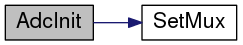Here is the caller graph for this function: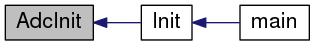UINT CalcAdcTemp ( UCHAR id )

calculate the temperature from specific ADC value in data array, specified by id

Parameters
 id for data array cell to get the temperature for
Returns
calculated temperature according to id

Definition at line 107 of file adc.c.

Referenced by main().

Here is the call graph for this function:Here is the caller graph for this function: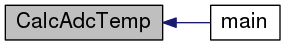UINT CalcSupplyVoltage ( UCHAR id )

Definition at line 126 of file adc.c.

Referenced by main().

Here is the call graph for this function: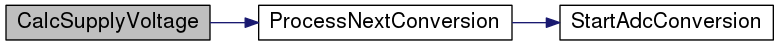Here is the caller graph for this function: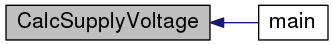ISR ( ADC_vect )

ADC interrupt service.

Parameters
 ADC_vect adc interrupt vector

Definition at line 41 of file adc.c.

Here is the call graph for this function: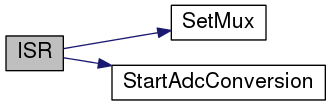void ProcessNextConversion ( void )

checks whether all converted values are processed and starts a new ADC conversion if the latter evaluates to TRUE

Definition at line 74 of file adc.c.

Referenced by CalcAdcTemp(), and CalcSupplyVoltage().

Here is the call graph for this function:Here is the caller graph for this function: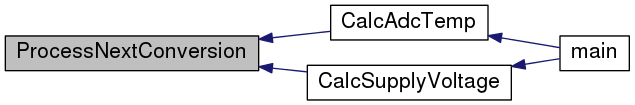void SetMux ( UCHAR ch )

set multiplexer thus switching between channels

Parameters
 ch adc channel id

Definition at line 97 of file adc.c.

References ADC_VREF_SEL, FIRST_ADC_INPUT, g_justMuxed, and TRUE.

Referenced by AdcInit(), and ISR().

Here is the caller graph for this function: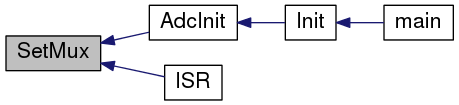void StartAdcConversion ( void )

start AD conversion, this will trigger the conversion of all selected channels via the interrupt

Definition at line 87 of file adc.c.

References ADC_SR, g_adcConverting, and TRUE.

Referenced by ISR(), and ProcessNextConversion().

Here is the caller graph for this function: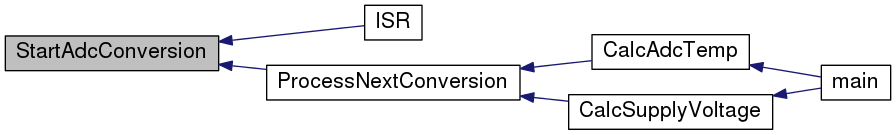## Variable Documentation

 UCHAR volatile g_adcConverting = FALSE

flag indicating conversion progress

Definition at line 14 of file adc.c.

Referenced by CalcAdcTemp(), CalcSupplyVoltage(), ISR(), and StartAdcConversion().

 UINT g_adcData[ADC_INPUTS]

list of converted data, according to selected inputs

Definition at line 10 of file adc.c.

Referenced by CalcAdcTemp(), CalcSupplyVoltage(), ISR(), and ProcessNextConversion().

 UCHAR volatile g_justMuxed = FALSE

flag indicating that the multiplexer has changed - throwing away the next conversion result

Definition at line 18 of file adc.c.

Referenced by ISR(), and SetMux().